# Math in Focus Grade 8 Chapter 2 Lesson 2.1 Answer Key Understanding Scientific Notation

Go through the Math in Focus Grade 8 Workbook Answer Key Chapter 2 Lesson 2.1 Understanding Scientific Notation to finish your assignments.

## Math in Focus Grade 8 Course 3 A Chapter 2 Lesson 2.1 Answer Key Understanding Scientific Notation

### Math in Focus Grade 8 Chapter 2 Lesson 2.1 Guided Practice Answer Key

Tell whether each number is written correctly in scientific notation. If it is incorrectly written, state the reason.

Question 1.
A Brazilian gold frog is about 9.6 • 10° millimeters long.
Given,
A Brazilian gold frog is about 9.6 • 10° millimeters long.
9.6 × 1 = 9.6 millimeters
The given statement is correct because the length of a Brazilian gold frog is about 9.6 mm

Question 2.
The wavelength of green light is about 4.15 • 10-7 meter.
Answer: The wavelength of green light is measured in a nanometer. Thus the above statement is incorrect.

Question 3.
Mars is approximately 0.2244 • 107 kilometers from the Sun.
Answer: The above statement is correct.

Write each number in scientific notation.

Question 4.
856.2
856.2 =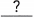Move the decimal pointplaces to the left and multiply by.
=Rewrite as a power of 10.
856.2 = 8562 • 10-1 Move the decimal point 1 place to the left and multiply by 8562 • 10-1
= 8562 • 10-1

Question 5.
0.06
6  • 10-2 Move the decimal point 2 places to the left and multiply 6 by 10-2

Write each number in standard form.

Question 6.
9 • 104
9 • 104 =Evaluate the power.
=Multiply by.
9 • 104
• indicates product of two numbers.
9 × 104
9 × 10000 = 90,000
9 multiply by 10000.

Question 7.
2.5 • 10-2
2.5 • 10-2 =Evaluate the power.
=Divide by.
2.5 • 10-2 = 2.5 • 10-2 Evaluate the power.
= 2.5 Divide by 1/100

Identify the lesser number in each pair of numbers. Justify your reasoning.

Question 8.
4.2 • 102 and 6.5 • 101<Compare the..
So,is the lesser number.
6.5 • 101  < 4.2 • 102 Compare the decimals.
6.5 • 101  < 4.2 • 102
So, 6.5 • 101 is the lesser number.

Question 9.
3.6 • 10-3 and 8.4 • 10-3
Theare the same.<Compare the.<.
So,is the lesser number.
The exponents are the same.
3.6 • 10-3 < 8.4 • 10-3
3.6 < 8.4
So, 3.6 is the lesser number

Complete.

Question 10.
An actor has 75,126 fans on a social network. A musician has 8.58 • 104 fans. Who has more fans on the social network?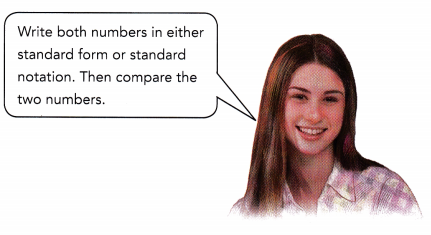Method 1
8.58 • 104 =>So, thehas more fans on the social network.

Method 2
75,126 =Compareand.
Theare the same.>Compare the coefficients.
So, thehas more fans on the social network.
Given,
An actor has 75,126 fans on a social network. A musician has 8.58 • 104 fans.
We have to find who has more fans on the social network.
When you multiply a decimal by a positive power of 10, the decimal point to the right.
8.58 • 104 = 858 × 10²
= 85800 fans
75,126 is less than 85800
Therefore we can say that the musician has more fans on the social network.

Question 11.
The average diameter of a type of round shaped bacteria is 0.0000037 meter. The spacing between two of these bacteria is 2.1 • 10-9 meter. Which length is shorter?
Given,
The average diameter of a type of round shaped bacteria is 0.0000037 meter.
The spacing between two of these bacteria is 2.1 • 10-9 meter.
0.0000037 meter can be written as
0.37 × 1/100000
0.37 × 10-5 meter
3.7 ×10 -6 meter
37 × 10-7 meter
2.1 • 10-9 meter is shorter than 37 • 10-7 meter

Question 12.
There are 5.816 • 104 spectators in a stadium watching a football game. In a theater, 1,150 people attend an opera. Which venue has more people?
Given,
There are 5.816 • 104 spectators in a stadium watching a football game.
In a theater, 1,150 people attend an opera.
We know that,
When you multiply a decimal by a positive power of 10, the decimal point to the right.
5.816 • 104 = 5.816  • 10000
= 5816 × 10
= 58160
1,150
58160 is greater than 1150
Thus the stadium watching a football game has more people than people attend an opera.

### Math in Focus Course 3A Practice 2.1 Answer Key

Tell whether each number is written correctly in scientific notation. If incorrectly written, state the reason.

Question 1
71 • 1022
71 • 1022
No the above number is not written in the scientific notation

Question 2.
8 • 10-2
8 • 10-2
= 8 × 1/100
= 0.08
No the above number is not written in the scientific notation

Question 3.
0.99 • 10-3
0.99 • 10-3
= 9.9 × 10-4
Yes the above number is written in the scientific notation

Question 4.
1.2 • 10-4
1.2 • 10-4
Yes the above number is written in the scientific notation

Write each number in scientific notation.

Question 5.
533,000
We can write the very large number in the standard form as the product of a number between 1 and 10 inclusive of 1 and an integer power of 10.
For the numbers greater than 1 we can use a positive exponent.
533 • 10³

Question 6.
327.8
We can write the very large number in the standard form as the product of a number between 1 and 10 inclusive of 1 and an integer power of 10.
For the numbers less than 1 we use a negative exponent.
3278 • 10-1

Question 7.
0.0034
We can write the very large number in the standard form as the product of a number between 1 and 10 inclusive of 1 and an integer power of 10.
For the numbers less than 1 we use a negative exponent.
34 • 10-4

Question 8.
0.00000728
We can write the very large number in the standard form as the product of a number between 1 and 10 inclusive of 1 and an integer power of 10.
For the numbers less than 1 we use a negative exponent.
728 • 10-8

Write each number in standard form.

Question 9.
7.36 • 103

Question 10.
2.431 • 104

Question 11.
5.27 • 10-2

Question 12.
4.04 • 10-4

Identify the lesser number in each pair of numbers. Justify your reasoning.

Question 13.
8.7 • 106 and 5.9 • 103
Answer: 5.9 • 103 < 8.7 • 106

Question 14.
4.8 • 103 and 9.6 • 107
Answer: 4.8 • 103 < 9.6 • 107

Question 15.
3.1 • 10-5 and 7.5 • 10-5
Answer: 3.1 • 10-5 < 7.5 • 10-5

Question 16.
6.9 • 10-3 and 4.3 • 10-3
Answer: 4.3 • 10-3 < 6.9 • 10-3

Question 17.
The table shows population data for some countries. Write each population ¡n scientific notation.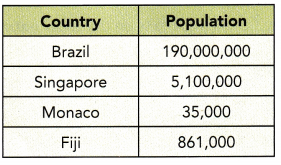From the above table, we can write the population in scientific notation.
Brazil – 190,000,000
19 • 107
Singapore – 5,100,000
51 • 105
Monaco – 35,000
35 • 103
Fiji – 861,000
861 • 103

Question 18.
Human blood contains red blood cells, white blood cells, and platelets. The table shows the approximate diameters of each of these cells in fractions of a meter. Write each diameter in scientific notation.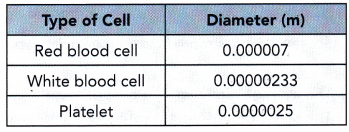Given,
Human blood contains red blood cells, white blood cells, and platelets.
The table shows the approximate diameters of each of these cells in fractions of a meter.
Red blood cell – 0.000007
= 7 • 10-6
White blood cell – 0.00000233
= 233 • 10-8
Platelet – 0.0000025
= 25 • 10-7

Question 19.
A praying mantis is approximately 15 centimeters long. A caterpillar is about 76 millimeters long.
a) Write both lengths in millimeters in scientific notation.
b) Write both lengths in centimeters in scientific notation.
How does writing the numbers using the same units help you compare them?
a) Write both lengths in millimeters in scientific notation.
Given,
A praying mantis is approximately 15 centimeters long.
15 × 10 millimeters
A caterpillar is about 76 millimeters long.
b) Write both lengths in centimeters in scientific notation.
A praying mantis is approximately 15 centimeters long.
76 × 10-1 centimeter

Question 20.
A technician reads and records the air pressure from several pressure gauges. The table shows each air pressure reading in pascals (Pa). A pascal is a unit used to measure the amount of force applied on a given area by air or other gases.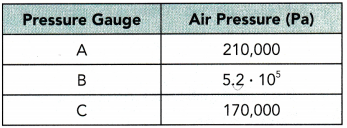a) Which pressure gauge has the greatest reading?
Pressure Gauge B has the greatest readings is 5.2 × 105 = 520000

b) Which pressure gauge has the lowest reading?
Pressure gauge C has the lowest reading is 170,000

c) The atmospheric pressure when these readings were made was 1.1 • 105 pascals. Which gauge(s) showed a reading greater than the atmospheric pressure?
All the gauges A, B, C are showed a greater reading than the atmospheric pressure.
210000 = 2.1 × 105
520000 = 5.2 × 105
170000 = 1.7 × 105

Question 21.
Math Journal The table shows some numbers written in standard form and in the equivalent scientific notation. Describe the relationship between the pair of variables described in a) and b).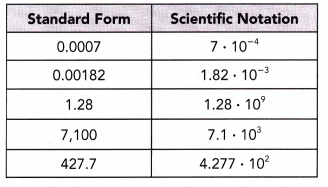a) The value of the positive number in standard form and the sign of the exponent when expressed in scientific notation.
When a number is written in scientific notation, the exponent tells you if the term is a large or a small number. A positive exponent indicates a large number and a negative exponent indicates a small number that is between 0 and 1.

b) The sign of the exponent when expressed in scientific notation and the direction the decimal point moves to express the number in standard form.
In order to go between scientific notation and decimals, the decimal point is moved to the number of spaces indicated by the exponent. A negative exponent tells you to move the decimal point to the right, while a positive exponent tells you to move it to the left.

Question 22.
Math Journal When visible light passes through a prism the light waves refract, or bend and the colors that make up the light can be seen. Each color has a different wavelength, as shown in the diagram, which is refracted to a different degree.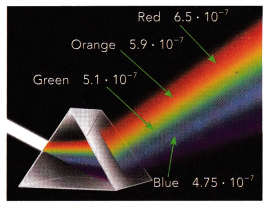a) Shorter wavelengths refract more than longer wavelengths. Which color of light wave shows the most refraction? Which color of light wave shows the least refraction?